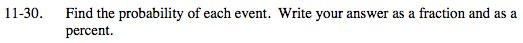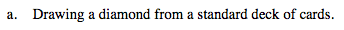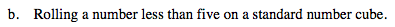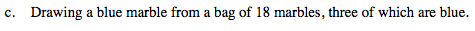Home > MC2 > Chapter 11 > Lesson 11.2.1 > Problem11-30

11-30.
1. Find the probability of each event. Write your answer as a fraction and as a percent. Homework Help ✎

1. Drawing a diamond from a standard deck of cards.

2. Rolling a number less than five on a standard number cube.

3. Drawing a blue marble from a bag of 18 marbles, three of which are blue.There are 13 diamond cards out of a deck of 52 cards.A standard number cube has faces numbered 1 through 6.

$\frac{4}{6} \text{ or } 66.67 \%$$\frac{3}{18} \text{ or } 16.67 \%$